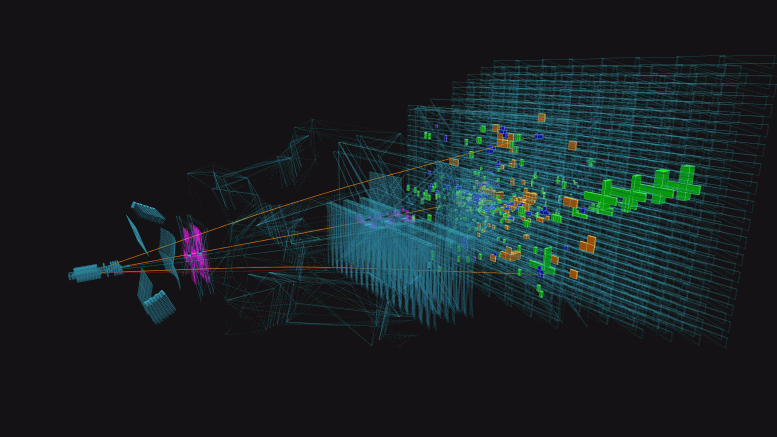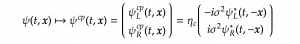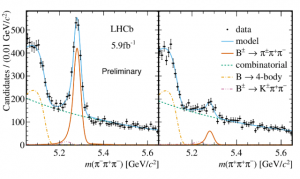# LHCb Reports New Observations of CP AsymmetriesThe Large Hadron Collider beauty (LHCb) studies the minor fundamental differences between matter and antimatter by studying the beauty quark. The major area of research at the LHCb is in observations of charge-parity (CP) violation, measuring the probabilities of matter/anti-matter decay mechanisms | Source: CERN

New results from the Large Hadron Collider beauty (LHCb) collaboration at CERN were presented at the Rencontres de Moriond Electroweak and a CERN seminar, showing a significant asymmetry between charmless decays of B± mesons to three-body states. The largest global asymmetry (measured between the decay probabilities) was identified in charged $$\text{B}$$ decays to two kaons and a pion. The standard matter decay $$(\text{B}^+ \to \pi ^+ \text{K}^+ \text{K}^- )$$ was favored over the antimatter decay $$(\text{B}^- \to \pi ^- \text{K}^+ \text{K}^- )$$ by approximately $$20 \%$$. This probability variation corresponds to a measured value of charge-parity (CP) asymmetry $$A_{\text{CP}} = -0.114$$, the largest occurrence of direct CP violation recorded.

C,P,T Symmetries

The conservation of particle physics theories under a parity transformation (a spatial inversion of coordinate signs), also known as parity symmetry (P-symmetry), remains consistent for all fundamental interactions of the Standard Model (SM), excluding weak interactions. For Dirac fermions, invariance under a parity inversion is dubbed chiral symmetry. However, this chiral symmetry was identified to be broken in various experiments of the mid-twentieth century, with the combination of charge-symmetry (C-symmetry) suggested as a solution to the violation. This combined symmetry, CP-symmetry, satisfied strong and electromagnetic interactions but were violated in rare instances of weak decays.The combined charge conjugation and parity transformation (CP) maps the Dirac spinor with the anti-particle field (inverse transpose of the Dirac spinor) and the complex charge-conjugate spinors. Note that the three spatial dimensions have been collapsed into a single spatial vector, reducing the four-vector into two components, with this $$\mathbf{x}$$ becoming negative as a result of the parity inversion | Typesetting: Dheeran Wiggins | Source: An Introduction to Quantum Field Theory (Peskin and Schroeder)

C-symmetry proposed that a charge conjugation transformation (the reversal of all fundamental charges, including electric charge and color) would yield a particle’s antiparticle. However, the unbalanced amounts of matter and antimatter within the universe suggest that a basic C-symmetry was violated during the conception of the universe, else the equal parts of matter and antimatter would have annihilated and resulted in a system balanced at net zero. Thus, a CP-violation through certain weak decays could account for the favoring of standard matter. An additional symmetry, the time-reversal symmetry (T-symmetry) led to the development of the CPT theorem, derived by manipulating rotational symmetries and their reflection equivalents in a Lorentz boost (using three spatial dimensions). This suggests that the inversion of parity (through an arbitrary point), charge, and momenta of all particles and antiparticles, would yield a universe with identically functioning physical laws. However, the weak CP-violations and matter-antimatter asymmetries suggest a related T-symmetry violation as well.

Because of limitations in observing T-asymmetries, primarily due to entropy flow in non-equilibrium states governed by the second law of thermodynamics, the experiments at facilities like the LHC focus on measuring direct CP violation.Histograms depicting the invariant mass of the three-pion final state decay of the $$\text{B}^-$$ and $$\text{B}^+$$ mesons, showing a distinct resonant peak at $$5.28 \text{ GeV}/c^2$$. There is a significant difference between the production at the resonant peak, favoring the antiparticle decay, potentially providing insight into the reason for these instances of CP asymmetries | Source: CERN

Direct CP-Violation

Direct violation of charge-parity symmetry involves measuring the branching ratios of various electroweak $$($$via the $$\text{W}^{\pm})$$ decays that show a favoring of matter production over antimatter production. These decays, though rare, provide immense insight into the mechanisms of CP asymmetry within the Standard Model. In addition to the favoring of the $$\text{B}^+ \to \pi ^+ \text{K}^+ \text{K}^-$$ decay, the LHCb detector also showed an immense presence of CP asymmetry within a subset of $$\chi _{\text{hc}}^0$$ meson non-final state data sets. This meson, formed by a charm and anticharm quark pair, was produced in the antimatter $$\text{B}^-$$ decays nearly seven times as frequently in comparison to its positively charged counterpart. Intermediate short-lived particles such as the $$\chi _{\text{hc}}^0$$ show differing invariant mass resonances in the three-pion final state histograms.

The process of disentangling the momenta of the final state particles (in this case, kaons, and pions) to determine the intermediate decay channels, provides significant insight into the specifics of the CP-violating decays. This analysis, dubbed “phase space analysis,” takes immense processing power and complex algorithms formed by teams of data-analytic physicists part of the LHCb collaboration. The results showing increased production of intermediate $$\chi _{\text{hc}}^0$$ mesons for the antiparticle decays provides physicists with additional information regarding the asymmetric decays, giving way to a potential theoretical explanation for the imbalances beyond the SM.

Future Run-3 at the LHCb detector, after upgrades to the LHC and the detector itself, will serve to solidify the results of this CP-violation, with the hope of further probing the reason for the imbalanced decay channels.